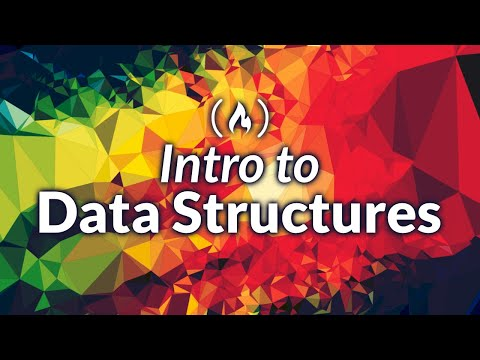Learn all about Data Structures in this lecture-style course. You will learn what Data Structures are, how we measure a Data Structures efficiency, and then hop into talking about 12 of the most common Data Structures which will come up throughout your Computer Science journey.

✏️ Course created by Steven from NullPointerExceptions. Check out their channel: https://www.youtube.com/channel/UCmWDlvMYYEbW42B8JyxFBcA

⭐️ Script, Visuals, and Sources ⭐️

⭐️ Links as They Appear ⭐️
🔗 An Introduction to Programming: https://www.youtube.com/watch?v=zOjov-2OZ0E
🔗 Binary Search Explanation: https://youtu.be/zOjov-2OZ0E?t=5566
🔗 Linear Search Explanation: https://youtu.be/zOjov-2OZ0E?t=5457
🔗 Introduction to Object Oriented Programming: https://www.youtube.com/watch?v=3ycztbPPOlc
🔗 Recursion Explanation: https://youtu.be/zOjov-2OZ0E?t=5787
🔗 Article explaining Backed Data Structures: https://tinyurl.com/y3fs9lt7

⭐️ Course Contents ⭐️
💻 (00:00) Introduction
⌨️ (01:06) Timestamps
⌨️ (01:23) Script and Visuals
⌨️ (01:34) References + Research
⌨️ (01:56) Questions
⌨️ (02:12) Shameless Plug
⌨️ (02:51) What are Data Structures?
⌨️ (04:36) Series Overview
💻 (06:55) Measuring Efficiency with BigO Notation
⌨️ (09:45) Time Complexity Equations
⌨️ (11:13) The Meaning of BigO
⌨️ (12:42) Why BigO?
⌨️ (13:18) Quick Recap
⌨️ (14:27) Types of Time Complexity Equations
⌨️ (19:42) Final Note on Time Complexity Equations
💻 (20:21) The Array
⌨️ (20:58) Array Basics
⌨️ (22:09) Array Names
⌨️ (22:59) Parallel Arrays
⌨️ (23:59) Array Types
⌨️ (24:30) Array Size
⌨️ (25:45) Creating Arrays
⌨️ (26:11) Populate-First Arrays
⌨️ (28:09) Populate-Later Arrays
⌨️ (30:22) Numerical Indexes
⌨️ (31:57) Replacing information in an Array
⌨️ (32:42) 2-Dimensional Arrays
⌨️ (35:01) Arrays as a Data Structure
⌨️ (42:21) Pros and Cons
💻 (43:33) The ArrayList
⌨️ (44:42) Structure of the ArrayList
⌨️ (45:19) Initializing an ArrayList
⌨️ (47:34) ArrayList Functionality
⌨️ (49:30) ArrayList Methods
⌨️ (53:57) Remove Method
⌨️ (55:33) Get Method
⌨️ (55:59) Set Method
⌨️ (56:57) Clear Method
⌨️ (57:30) toArray Method
⌨️ (59:00) ArrayList as a Data Structure
⌨️ (1:03:12) Comparing and Contrasting with Arrays
💻 (1:05:02) The Stack
⌨️ (1:05:06) The Different types of Data Structures
⌨️ (1:05:51) Random Access Data Structures
⌨️ (1:06:10) Sequential Access Data Structures
⌨️ (1:07:36) Stack Basics
⌨️ (1:09:01) Common Stack Methods
⌨️ (1:09:45) Push Method
⌨️ (1:10:32) Pop Method
⌨️ (1:11:46) Peek Method
⌨️ (1:12:27) Contains Method
⌨️ (1:13:23) Time Complexity Equations
⌨️ (1:15:28) Uses for Stacks
💻 (1:18:01) The Queue
⌨️ (1:18:51) Queue Basics
⌨️ (1:20:44) Common Queue Methods
⌨️ (1:21:13) Enqueue Method
⌨️ (1:22:20) Dequeue Method
⌨️ (1:23:08) Peek Method
⌨️ (1:24:15) Contains Method
⌨️ (1:25:05) Time Complexity Equations
⌨️ (1:27:05) Common Queue Uses
⌨️ (1:33:55) Adding and Removing Information
⌨️ (1:41:28) Time Complexity Equations
⌨️ (1:48:44) Visualization
⌨️ (1:50:56) Adding and Removing Information
⌨️ (1:58:30) Time Complexity Equations
⌨️ (1:59:06) Uses of a Doubly-LinkedList
💻 (2:00:21) The Dictionary
⌨️ (2:01:15) Dictionary Basics
⌨️ (2:02:00) Indexing Dictionaries
⌨️ (2:02:40) Dictionary Properties
💻 (2:05:53) Hash Table Mini-Lesson
⌨️ (2:13:26) Time Complexity Equations
💻 (2:16:39) Trees
⌨️ (2:16:55) Introduction to Hierarchical Data
⌨️ (2:18:54) Formal Background on the Tree
⌨️ (2:20:03) Tree Terminology and Visualization
⌨️ (2:25:08) Different types of Trees
⌨️ (2:28:07) Uses for the Tree
💻 (2:29:00) Tries
⌨️ (2:29:50) Trie Basics
⌨️ (2:30:41) Trie Visualization
⌨️ (2:34:33) Flagging
⌨️ (2:35:15) Uses for Tries
💻 (2:38:25) Heaps
⌨️ (2:38:51) Heap Basics
⌨️ (2:39:19) Min-Heaps
⌨️ (2:40:07) Max-Heaps
⌨️ (2:40:59) Building Heaps
⌨️ (2:44:20) Deleting from Heaps
⌨️ (2:46:00) Heap Implementations
💻 (2:48:15) Graphs
⌨️ (2:49:25) Graph Basics
⌨️ (2:52:04) Directed vs. Undirected Graphs
⌨️ (2:53:45) Cyclic vs. Acyclic Graphs
⌨️ (2:55:04) Weighted Graphs
⌨️ (2:55:46) Types of Graphs
💻 (2:58:20) Conclusion
💻 (2:58:43) Shameless Plug